﻿ A Critical Appraisal of Modern Engineering Science, and the Changes Required by the Appraisal ConclusionsPublications are Open
Access in this journal
Article Versions
Export Article
• Normal Style
• MLA Style
• APA Style
• Chicago Style
Research Article
Open Access Peer-reviewed

### A Critical Appraisal of Modern Engineering Science, and the Changes Required by the Appraisal Conclusions

American Journal of Mechanical Engineering. 2020, 8(4), 144-153. DOI: 10.12691/ajme-8-4-1
Received September 22, 2020; Revised October 24, 2020; Accepted November 01, 2020

### Abstract

Until the nineteenth century, engineering science was founded on a view of dimensional homogeneity that required the following: • Parameters must not be multiplied or divided. • Dimensions must not be assigned to numbers. • Equations must be dimensionless. This view made it impossible to create equations such as the laws of modern engineering science. Modern engineering science is founded on Fourier’s radically different nineteenth century view of dimensional homogeneity. His view allows the following, and makes it possible to create the laws of modern engineering science: • Parameters may be multiplied or divided. • Dimensions may be assigned to numbers. • Equations may or may not be dimensionless. Fourier did not prove the validity of his radically different view of dimensional homogeneity. He merely stated that his view of dimensional homogeneity “is the equivalent of the fundamental lemmas which the Greeks have left us without proof”. Presumably, his colleagues accepted his unproven view because he solved problems they were unable to solve. A critical appraisal of Fourier’s unproven view of dimensional homogeneity results in the following conclusions: • Parameters cannot rationally be multiplied or divided. Only the numerical values of parameters can rationally be multiplied or divided. • Dimensions cannot rationally be assigned to numbers. If dimensions could be assigned to numbers, any equation could be regarded as dimensionally homogeneous. • Equations are inherently dimensionless and dimensionally homogeneous because symbols in parametric equations can rationally represent only numerical value. The changes required by the appraisal conclusions result in a much simpler engineering science because parameters such as material modulus and heat transfer coefficient are abandoned. They are abandoned because problems are readily solved without them, and because when dealing with nonlinear behavior (as in the inelastic region and in various forms of convection heat transfer), they are extraneous variables that greatly complicate solutions. Examples in the text demonstrate how to solve problems without using parameters such as material modulus or heat transfer coefficient.

### 1. Introduction

Until the 19th century, engineering science was founded on a view of dimensional homogeneity that made it impossible to create equations such as the laws of modern engineering science. Fourier 1 conceived a view of dimensional homogeneity that made it possible to create equations such as the laws of modern engineering science, and he is generally credited with the modern view of dimensional homogeneity.

Fourier made no effort to prove the validity of his view of dimensional homogeneity. He merely stated that his view of dimensional homogeneity “is the equivalent of the fundamental lemmas which the Greeks have left us without proof”.

Because Fourier’s unproven view of dimensional homogeneity has been the foundation of engineering science for 200 years, this paper critically appraises his view of dimensional homogeneity, and describes changes in modern engineering science required by the appraisal conclusions.

### 2. The Multiplication or Division of Parameters was irrational until the Nineteenth Century

Until the nineteenth century, the generally accepted view of dimensional homogeneity was based on the following:

• With one exception, parameters must not be multiplied or divided. The one exception was that a parameter may be divided by itself. For example, the number of feet may be divided by the number of feet, and the number of seconds may be divided by the number of seconds, but the number of feet must not be divided by the number of seconds.

• Dimensions must not be assigned to numbers.

• Equations must be dimensionless.

The requirement that parameters must not be multiplied or divided made it impossible to generate equations such as the laws of modern engineering science. Because equations had to be dimensionless, each term in an equation had to be a ratio of the same parameter. The following verbal equation by Galileo 2 is typical. It is dimensionless and dimensionally homogeneous because each term is a ratio of the same parameter.

If two moveables are carried in equable motion, the ratio of their speeds will be compounded from the ratio of spaces run through and from the inverse ratio of times.

Because it was (and still is) assumed that proportions need not be dimensionally homogeneous, engineering laws were generally in the form of inhomogeneous proportions rather than equations. That is why the original version of Newton’s second law of motion 3 is not Eq. (1). It is inhomogeneous Proportion (2). And Hooke’s law 4 is not an equation. It is inhomogeneous Proportion (3).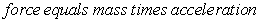(1)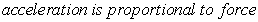(2)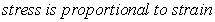(3)

It is quite certain that Newton and Hooke were sufficiently knowledgeable to transform Proportions (2) and (3) to equations. They did not transform them because the equations that would have resulted would have violated the view of dimensional homogeneity that prevailed until the nineteenth century.

### 3. Fourier’s View of Dimensional Homogeneity

Early in the nineteenth century, Fourier performed experiments in heat transfer by convection and conduction. From his data, Fourier concluded that, if heat transfer is by steady-state forced convection to atmospheric air, heat flux q is always proportional to temperature difference ΔT, as in Proportion (4).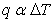(4)

Proportion (4) would have satisfied Galileo, Hooke, and Newton, but it did not satisfy Fourier. Fourier wanted an equation, and it had to be dimensionally homogeneous. The transformation from Proportion (4) to an equation results in Eq. (5) in which c is a number.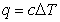(5)

Equation (5) is not dimensionally homogeneous because c is dimensionless, and the dimension of q is not equal to the dimension of ΔT. Fourier recognized that Eq. (5) could be transformed to a dimensionally homogeneous equation only if the then current view of dimensional homogeneity were replaced by a view in which dimensions can be assigned to numbers, and parameters can be multiplied and divided. Consequently Fourier conceived a radically different view of dimensional homogeneity in which dimensions can be assigned to numbers, and parameters can be multiplied and divided. Fourier described his view of dimensional homogeneity in the following:

. . . every undetermined magnitude or constant has one dimension proper to itself, and the terms of one and the same equation could not be compared, if they had not the same exponent of dimension. . . this consideration is derived from primary notions on quantities; for which reason, in geometry and mechanics, it is the equivalent of the fundamental lemmas which the Greeks have left us without proof. Fourier 1.

Even though Fourier’s nearly 500 page treatise (The Analytical Theory of Heat) is predicated on the validity of his view of dimensional homogeneity, Fourier:

• Made no effort to prove that his view of dimensional homogeneity is valid.

• Did not include the fundamental lemmas (axioms) which he alleged would establish the validity of his view.

• Did not cite a reference in which the fundamental lemmas could be found.

Presumably, Fourier’s colleagues accepted his unproven view of dimensional homogeneity because he solved problems they were unable to solve.

### 4. The Importance of Fourier’s Unproven View of Dimensional Homogeneity

Fourier’s unproven view of dimensional homogeneity is important because, for 200 years, it has been the foundation of engineering science. His view of dimensional homogeneity is the only reason it has been considered rational to:

• Assign dimensions to numbers.

• Multiply and divide parameters.

• Generate equations that describe how parameters are related.

### 5. How Fourier Transformed Inhomogeneous Eq. (5) to Homogeneous Eq. (6)

In accordance with his view that dimensions may rationally be assigned to numbers, Fourier transformed Eq. (5) from inhomogeneous to homogeneous by assigning to number c the symbol h and the dimension of q/ΔT. The result was the dimensionally homogeneous Eq. (6), Fourier’s law of steady-state forced convection heat transfer to atmospheric air.

In much of the nineteenth century, Equation (6) was a law. It stated that, if heat transfer is by steady-state forced convection to atmospheric air, q is always proportional to ΔT, and h is always a proportionality constant.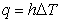(6)

### 6. Fourier’s Definition of h

Fourier’s 5 definition of h is:

We have taken as the measure of the external conducibility of a solid body a coefficient h, which denotes the quantity of heat which would pass, in a definite time (a minute), from the surface of this body, into atmospheric air, supposing that the surface had a definite extent (a square metre), that the constant temperature of the body was 1, and that of the air 0, and that the heated surface was exposed to a current of air of a given invariable velocity.

In other words, if heat is transferred from a heated surface to atmospheric air, and if there is a steady-state current of air over the surface, h is the quantity of heat transferred per minute per square metre (ie heat flux q) per boundary layer temperature difference of one degree Reamur (ie ΔT)—ie Fourier defined h to be the symbol for q/ΔT.

In modern engineering science, h is still defined to be the symbol for q/ΔT, but the modern definition does not specify the heat transfer fluid, and does not require forced convection over the heat transfer surface.

### 7. Why Eq. (6) Ceased to be an Equation/Law Sometime Near the Beginning of the Twentieth Century

Sometime near the beginning of the twentieth century, it was decided to apply Eq. (6) to nonlinear forms of heat transfer such as natural convection, condensation, and boiling. Because Eq. (6) is a proportional equation, it cannot describe nonlinear behavior. Therefore, when it was decided to apply Eq. (6) to nonlinear phenomena, Eq. (6) ceased to be an equation/law because it no longer described the relationship between q and ΔT in a general way. Eq. (6) still defined h to be a symbol for q/ΔT, but Eq. (6) was transformed from an equation/law to a definition in the inappropriate form of a proportional equation.

In order to be rigorously correct, Eq. (6) should have been abandoned when it began to be applied to nonlinear forms of heat transfer, and it should have been replaced by Definition (6a).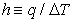(6a)

Definition (6a) correctly indicates that:

• The relationship between q and ΔT may be proportional, linear, or nonlinear.

h may be a constant or a variable.

h is the symbol for q/ΔT.

However, Eq. (6) was not abandoned. It was retained, and American heat transfer texts generally refer to Eq. (6) as “Newton’s law of cooling”. (Equation (6) cannot be Newton’s law of cooling because cooling is a transient phenomenon, and Eq. (6) is a steady-state equation.)

### 8. How the Modern View of Dimensional Homogeneity Differs from Fourier’s View, and How the Difference Impacts Modern Engineering Science

Fourier is generally credited with the modern view of dimensional homogeneity. However, the modern view differs from Fourier’s view in one very important way. Langhaar 6 states:

Dimensions must not be assigned to numbers, for then any equation could be regarded as dimensionally homogeneous.

Therefore parameters such as h and E, and laws such as Eqs. (7), (8), and (9), are irrational because they were created by assigning dimensions to numbers, in violation of the modern view that dimensions must not be assigned to numbers.(7)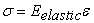(8)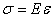(9)

### 9. What q = hΔT and σ = Eε Really Mean

Because h is defined to be a symbol for q/ΔT, Eqs. (7) and (7a) are identical. Therefore Eq. (7) really means that, given the value of ΔT, the value of q is determined by first determining the value of q/ΔT (ie the value of h), then multiplying q/ΔT by the given value of ΔT.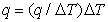(7a)

Because E is defined to be a symbol for σ/ε, Eqs. (9) and Eq. (9a) are identical. Therefore Eq. (9) really means that, given the value of ε, the value of σ is determined by first determining the value of σ/ε (ie the value of E), then multiplying σ/ε by ε.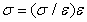(9a)

### 10. A Mathematical Analog of Modern Correlations Used to Describe Convection Heat Transfer and Stress/Strain

x,y data are generally correlated in the form y = f{x} because this equation:

• Can be solved in a direct manner if x is given, or if y is given.

Always concerns only two variables (x and y).

Reveals the relationship between x and y.

x,y data can also be correlated in the form (y/x) = f{x}. This methodology is seldom used because, if y is not proportional to x, this equation:

Cannot be solved in a direct manner if y is given and x is to be determined.

Always concerns three variables (x, y, and y/x).

Masks the relationship between x and y.

In modern convection heat transfer, analogs of (y/x) = f{x} are used to correlate q and ΔT data, resulting in (q/ΔT){ΔT} correlations—ie h{ΔT} correlations.

In modern stress/strain, analogs of (y/x) = f{x} are used to correlate σ,ε data, resulting in (σ/ε){ε} correlations—ie E{ε} correlations.

### 11. Why the Multiplication or Division of Parameters is irrational

Until the nineteenth century, scientists and engineers correctly considered it irrational to multiply or divide parameters. Their view is validated by the following:

• Multiplication is repeated addition. Six times seven means add seven six times. Therefore meters times kilograms means add kilograms meter times. Because “meter times” has no meaning, it is not possible to multiply meters times kilograms. Therefore it is not possible to multiply dimension units.

• Twelve divided by four means how many fours are in twelve. Therefore meters divided by seconds means how many seconds are in meters. Because “how many seconds are in meters” has no meaning, it is not possible to divide meters by seconds. Therefore it is not possible to divide dimension units.

The quantification of parameters requires the specification of their numerical values and their dimension units. Therefore the multiplication or division of parameters requires the multiplication or division of their numerical values and the multiplication or division of their dimension units.

Because the quantification of parameters includes dimension units, and because it is not possible to multiply or divide dimension units, it is not possible to multiply or divide parameters. Only the numerical values of parameters can rationally be multiplied or divided.

### 12. Why Parameter Symbols in Proportions and Equations must Represent only Numerical Values

• Pigs cannot be proportional to airplanes because pigs and airplanes are different things, and different things cannot be proportional. However, the number of pigs can be proportional to the number of airplanes.

• Stress cannot be proportional to strain because stress and strain are different things, and different things cannot be proportional. However, the numerical value of stress can be proportional to the numerical value of strain.

• Equations cannot rationally describe how pigs and airplanes are related because pigs and airplanes are different things, and different things cannot be related. However, equations can rationally describe how the number of pigs is related to the number of airplanes. (For example, the number of pigs that can be transported by air can equal a constant times the number of available Boeing 747 airplanes.)

• Equations cannot rationally describe how stress and strain are related because stress and strain are different things, and different things cannot be related. However, equations can rationally describe how the numerical value of stress is related to the numerical value of strain.

Parameter symbols in proportions and equations must represent only numerical values because proportions and equations cannot describe how different parameters (such as stress and strain or heat flux and temperature difference) are related. Proportions and equations can only describe how the numerical values of different parameters are related. If a parametric equation is quantitative, the dimension units that underlie parameter symbols must be specified in an accompanying nomenclature.

### 13. How Equations Are Like Charts

Charts cannot describe how parameters are related. Charts can describe only how the numerical values of parameters are related. If a chart is quantitative, the dimension units that underlie numerical values must be specified on the chart, or in an accompanying nomenclature.

Equations cannot describe how parameters are related. Equations can describe only how the numerical values of parameters are related. If an equation is quantitative, the dimension units that underlie numerical values must be specified in an accompanying nomenclature.

### 14. How the Appraisal Conclusions Impact Modern Engineering Science

The appraisal conclusions impact modern engineering science in the following ways:

• Because the modern view of dimensional homogeneity requires that dimensions not be assigned to numbers, all laws and parameters created by assigning dimensions to numbers, such as Eqs. (7), (8), and (9), and parameters such as E and h, are irrational, and must be abandoned.(7)(8)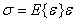(9)

• Parameters cannot be multiplied or divided because their dimension units cannot be multiplied or divided. Only the numerical values of parameters can be multiplied or divided.

• Parameter symbols in equations must represent only numerical values because equations can rationally describe only how the numerical values of parameters are related.

• Because parameter symbols in equations must represent only numerical values, all rational parametric equations are inherently dimensionless and dimensionally homogeneous.

• If parameter symbols represent only numerical values, and a parametric equation is quantitative, the dimension units that underlie parameter symbols must be specified in an accompanying nomenclature.

### 15. Rational Engineering Laws

Rational engineering laws must:

• Have parameter symbols that represent only numerical value.

• Identify the primary parameters.

• Describe exactly the same behavior described by data.

Always apply.

### 16. A Rational Law of Stress and Strain

Data indicate that the numerical value of stress is always a function of the numerical value of strain, and the function may be proportional, linear, or nonlinear. Therefore the law of stress and strain must be Eq. (10) because it:

• Has parameter symbols that represent only numerical value.

• Identifies the primary parameters.

• Describes exactly the same behavior described by data—ie allows that the behavior may be proportional, linear, or nonlinear.

Always applies in both the elastic and inelastic regions.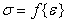(10)

Equation (10) states that the numerical value of stress is always a function of the numerical value of strain, and the function may be proportional, linear, or nonlinear.

### 17. Why the Proposed Law of Stress and Strain, Eq. (10), Has Little Impact on the Solution of Problems that Concern the Elastic Region

Equations (8) and (11) apply in the elastic region. Equation (8) is Young’s law, Eq. (11) is the elastic region form of Eq. (10).(8)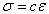(11)

Because Eelastic is a constant, both Eqs. (8) and Eq. (11) have only two variables, σ and ε. Therefore elastic region problems are easy to solve whether Eq. (8) or (11) is used in the solution. The only difference between the two solutions is that Eelastic has numerical value and dimension, whereas c has only numerical value.

### 18. Why the Proposed Law of Stress and Strain, Eq. (10), Greatly Simplifies the Solution of Most Problems in the Inelastic Region

Equations (9) and (10) apply in both the elastic and inelastic regions. In the inelastic region, E{ε} and Eq. (10) are so highly nonlinear that they are generally described graphically.(9)(10)

In the inelastic region:

• Eq. (9) always has three variables because E{ε} is a variable.

• Eq. (10) always has only two variables.

The proposed law greatly simplifies the solution of most inelastic problems because Eq. (9) always has three variables, whereas the proposed law, Eq. (10), always has two variables.

### 19. A Simple Problem that Demonstrates that not Using Modulus Greatly Simplifies the Solution of Most Problems in the Inelastic Region

19.1. Statement of Problem 1 Using E—ie Using σ /ε

If the stress is 40,000 kg/cm2, what is the strain? Use Figure 1.

• Figure 1. Modulus vs strain curve
19.2. Analysis of Problem 1 using Modulus.

Figure 1 must be read in an indirect manner because ε and σ are combined in E, the ratio σ/ε.

19.3. Statement of Problem 1 without Using E—ie without Using σ /ε.

If the stress is 40,000 kg/cm2, what is the strain? Use Figure 2.

19.4. Analysis and Solution of Problem 1 without Using Modulus

Inspection of Figure 2 indicates that, if the stress is 40,000 kg/cm2, the strain is .0013, .0037, or .0066. The problem statement does not include sufficient information to determine a unique solution.

• Figure 2. Stress vs strain curve
19.5. Conclusions Based on Problem 1

The solution of Problem 1 without using modulus:

• Is so simple it is easily solved by someone who knows nothing about stress and strain and nothing about mathematics.

• Requires nothing more than reading Figure 2.

• Is obtained in about 10 seconds because Figure 2 can be read directly. Figure 2 can be read directly because it is in the desirable form σ = f{ε}.

• Is so simple there is little likelihood of error.

The solution of Problem 1 using modulus:

• Is not simple and cannot be solved by someone who knows nothing about stress and strain and nothing about mathematics.

• Requires that Figure 1 be read indirectly because the chart is in the undesirable form E = f {ε}—ie the undesirable form (σ/ε) = f {ε}.

• Takes much longer and is much more difficult than the solution without using modulus because reading Figure 1 indirectly is much more difficult than reading Figure 2 directly.

• Has a much greater likelihood of error because Figure 1 must be read indirectly, and because the problem does not have a unique solution.

Although Problem 1 is trivial, it validates the conclusion that the solution of most inelastic problems is much simpler if modulus is not used.

### 20. A Rational Law of Convection Heat Transfer

Data indicate that the numerical value of convection heat flux is always a function of the numerical value of boundary layer temperature difference, and the function may be proportional, linear, or nonlinear. Therefore the law of convection heat transfer must be Eq. (12) because it:

• Has parameter symbols that represent only numerical value.

• Identifies the primary parameters.

• Describes exactly the same behavior described by data—ie allows that the behavior may be proportional, linear, or nonlinear.

Always applies.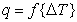(12)

Equation (12) states that the numerical value of heat flux is always a function of the numerical value of boundary layer temperature difference, and the function may be proportional, linear, or nonlinear.

### 21. How h Is Eliminated in Equations that Explicitly or Implicitly include h.

To eliminate h in equations that explicitly or implicitly include h, replace h and k/t with q/ΔT, then separate q and ΔT. For example, Eq. (13) is used to analyze heat transfer between two fluids separated by a flat wall.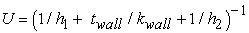(13)

To eliminate h, k, and U in Eq. (13):

• Substitute q/ΔTtotal for U. Substitute q/ΔT1 for h1 and q/ΔT2 for h2.

• Substitute q/ΔTwall for kwall/twall.

• Separate q and ΔT, resulting in Eq. (14).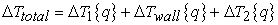(14)

Equations (13) and (14) are identical—they differ only in form. Therefore, any problem that can be solved using Eq. (13) and h can also be solved using Eq. (14) without h.

Equation (15) is a heat transfer coefficient correlation used in the analysis of forced convection heat transfer. To eliminate h, replace Nu with qD/ΔTk, then separate q and ΔT, resulting in Eq. (16a) or (16b).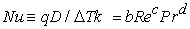(15)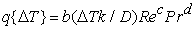(16a)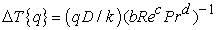(16b)

### 22. Why the Proposed Law of Convection Heat Transfer, Eq. (12), Greatly Simplifies the Solution of most Problems that Concern Nonlinear Behavior—ie Problems that Concern Natural Convection, Condensation, or Boiling

Equations (7) and (12) apply to all forms of convection heat transfer.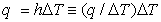(7)(12)

When applied to problems that concern nonlinear behavior, Eq. (7) has three variables (q, ΔT, and q/ΔT (ie h)), whereas Eq. (12) has only two variables (q and ΔT). The proposed law, Eq. (12), greatly simplifies the solution of most problems that concern nonlinear behavior because Eq. (7) has three variables whereas Eq. (12) has only two variables.

### 23. Problem 2 A Simple Problem that Demonstrates that not Using Heat Transfer Coefficients Greatly Simplifies the Solution of Most Problems that Concern moderately Nonlinear Thermal Behavior

23.1. Statement of Problem 2 Using h (ie Using q/ΔT)

Use h (ie q/ΔT) to determine the heat flux through a flat wall that separates Fluids 1 and 2.

23.2. Given, Problem 2 Using h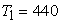(17)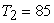(18)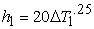(19)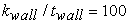(20)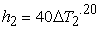(21)
23.3. Analysis, Problem 2 Using h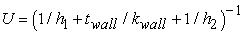(22)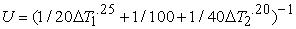(23)

Solve Eq. (23) to determine U, then multiply U by ΔTtotal.

23.4. Statement of Problem 2 without Using h (ie without Using q/ΔT)

Without using h, determine the heat flux through a flat wall that separates two fluids.

23.5. Given, Problem 2 without Using h(24)(25)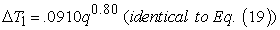(26)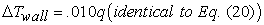(27)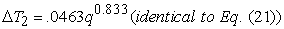(28)
23.6. Analysis and Solution, Problem 2 without Using h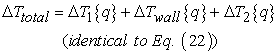(29)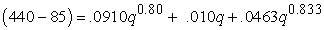(30)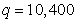(31)
23.7. Conclusions Based on Problem 2

Problem 2 demonstrates that the solution of most moderately nonlinear problems is much more difficult if h is used in the solution. Note that:

• Equation (30) has only one unknown variable.

• Using Excel and trial-and-error methodology, Eq. (30) can be solved in about a minute by someone who knows nothing about heat transfer and nothing about mathematics.

• Equation (23) has three unknown variables (U, ΔT1, and ΔT2).

• Equation (23) can be solved only by someone who knows a good deal about heat transfer and a good deal about mathematics.

• In order to solve Eq. (23), it is necessary to:

○ Find two more equations that apply to the problem.

○ Solve the three equations simultaneously to determine q/ΔTtotal—ie to determine U.

○ Multiply q/ΔTtotal times ΔTtotal to determine q.

• It takes much longer than a minute to solve Eq. (23).

• There is a much greater likelihood of error in the solution of Eq. (23).

### 24. Problem 3 How to Solve Thermal Stability Problems without Using h.

24.1. Statement of Problem 3

A vented pool boiler has a horizontal boiler plate, a boiling fluid (Fluid 2) above the boiler plate, and a heat source fluid (Fluid 1) below the boiler plate. Without using h, describe:

• How to determine thermally stable and thermally unstable boiler operating points.

• Undamped oscillations that can occur.

• How to design the boiler so that undamped oscillations cannot occur.

• How to design the boiler so that it can operate stably at all points in the “transition boiling region”.

24.2. Given, Problem 3

• The thermal behavior of the boiling interface (qout) is qualitatively described in Figure 3. The y axis is labeled qout because Figure 3 concerns heat flux out of the boiling interface.

• The temperature of the boiler heat source (Fluid 1) is T1.

• The temperature of the boiling fluid (Fluid 2) is Tsat. Tsat is fixed because the boiler is vented.

There is no boiling in Region 1. Heat transfer is by natural convection from the boiler plate to Fluid 2, and by evaporation at the surface of Fluid 2.

• Figure 3. The thermal behavior of the boiling interface in Problem 3
24.3. How to Determine Thermally Stable and Thermally Unstable Operating Points, Problem 3

To determine operating points, determine qin{Tbi} (the relationship between heat flux into the boiling interface and the temperature of the boiling interface), and plot it on Figure 3. For example, if qin{Tbi} is proportional to (T1 - Tbi), then qin{Tbi} is a straight line of negative slope on Figure 3, and the line meets the x axis at T1 = Tbi. Operating points are at intersections of qin{Tbi} and qout{Tbi}.

Determine the thermal stability at each intersection by comparing the slopes of the qin{Tbi} and qout{Tbi} lines. At thermally unstable operating points, the slope of the qin{Tbi} line is greater than the slope of the qout{Tbi} line. In other words, intersections are thermally unstable if Criterion (32) is satisfied: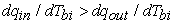(32)

Because the slopes of the qin{Tbi} lines are negative, all intersections at which the slope of the qout{Tbi} line is positive are thermally stable. Therefore Regions 2 and 4 are the only regions in which there might be thermally unstable intersections.

24.4. Undamped Oscillations that Can Occur

Undamped oscillations in heat flux and temperature will occur if:

• There is a single intersection between qin{Tbi} and qout{Tbi}.

• Criterion (32) is satisfied.

A single intersection in Region 2 would satisfy Criterion (32) because the slope of the qin{Tbi} line would necessarily be greater than the slope of the qout{Tbi} line. Because there is only one intersection and it is thermally unstable, the boiler would leave the intersection, and undamped oscillations would result. The undamped oscillations are described by the arrows in Figure 4. The arrows indicate that the boiler continually alternates between non-boiling on Line 1, and boiling on Line 3.

A single intersection in Region 4 would be thermally stable because the slope of the qin{Tbi} line would necessarily be less than the slope of the qout{Tbi} line.)

• Figure 4. Undamped oscillations that can result in Region 2
24.5. How to Design the Boiler so that Undamped Oscillations cannot Occur

In order to ensure that undamped oscillations cannot occur, the boiler must be designed so that qin{Tbi} also intersects Lines 1 and 3 whenever it intersects Line 2. If qin{Tbi} intersects Lines 1 and 3 whenever it intersects Line 2:

• Intersections of qin{Tbi} and Lines 1, 2, and 3 are thermally stable.

• The boiler operates stably at intersections with Lines 1 and 3, but hysteresis prevents the boiler from operating at the intersection with Line 2. Hysteresis results because the slopes of qin{Tbi} lines that pass through the intersection of Lines 1 and 2 and the intersection of lines 2 and 3 are necessarily more negative than the slope of Line 2.

24.6. How to Design the Boiler to Operate Stably at all Points in the “transition boiling region” (ie Line 4 in Figure 3 and Figure 4)

In order to design the boiler to operate stably at all points in the “transition boiling region” (ie Line 4), it must be designed so that dqin/dTbi is less than dqout/dTbi at all points on Line 4. (If dqin/dTbi were greater than dqout/dTbi at a point on Line 4, there would necessarily also be intersections with Lines 3 and 5. The boiler would be thermally unstable at the intersection with Line 4, but undamped oscillations would not result because if the boiler was initially at the Line 4 intersection, it would automatically transition to the intersection with Line 3 or Line 5 where it would continue to operate in a thermally stable manner.)

24.7. Solution of Problem 3 Using h{ΔT} Methodology

Heat transfer texts generally do not describe how to use h{ΔT} methodology to solve thermal stability problems. It seems more than likely that the solution of Problem 3 using h{ΔT} methodology would be much more difficult than the above solution using q{ΔT} methodology.

### 25. Conclusions

• Dimensions cannot rationally be assigned to numbers. If dimensions could rationally be assigned to numbers, any equation could be dimensionally homogeneous.

• Dimension units cannot rationally be multiplied or divided.

• Parameters cannot rationally be multiplied or divided because their dimension units cannot rationally be multiplied or divided.

• Equations cannot rationally describe how parameters are related because parameters cannot be related. Equations can describe only how the numerical values of parameters are related.

• If an equation is quantitative and parameter symbols are dimensionless, the dimension units that underlie parameter symbols must be specified in an accompanying nomenclature.

• Rational parametric equations are inherently dimensionless and dimensionally homogeneous because parameter symbols in equations must represent only numerical value.

• Engineering laws in the form of proportional equations should be replaced by analogs of Eq. (33) in which y and x are the numerical values of primary parameters such as stress and strain, or heat flux and temperature difference, etc.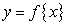(33)

• Laws that are analogs of Eq. (33) are true laws because:

○ They have parameter symbols that represent only numerical value.

○ They identify the primary parameters.

○ They describe exactly the same behavior described by data—ie they allow the behavior to be proportional, linear, or nonlinear.

○ They always apply.

• Equation (34) should replace Eq. (35), and Eq. (36) should replace Eq. (37).(34)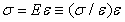(35)(36)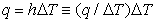(37)

• Parameters such as q/ΔT (ie h) and σ/ε (ie E) should be abandoned because they are unnecessary and undesirable. They are unnecessary because problems are readily solved without them. They are undesirable because, when dealing with problems that concern nonlinear behavior, they are extraneous variables that greatly complicate solutions.

The engineering science that results from abandoning parameters such as h (ie q/ΔT) and E (ie σ/ε) is much easier to learn and apply because there are fewer parameters that must be understood and applied, and because the primary parameters are not combined in ratios such as (q/ΔT) and (σ/ε) that greatly complicate the solution of nonlinear problems by making it impossible to solve them with the primary variables separated, the methodology preferred in mathematics.

### Nomenclature

Symbols

Note: The symbols may represent numerical value and dimension, or numerical value alone.

c arbitrary number

D diameter

E modulus, symbol for σ/ε, kg/cm2

h heat transfer coefficient, symbol for q/ΔT, W/m2K

k thermal conductivity, symbol for q/(dT/dx), W/mK

Nu Nusselt number

Pr Prandtl number

q heat flux, W/m2

Re Reynolds number

T temperature, C

t thickness, m

U overall heat transfer coefficient, symbol for q/ΔTtotal, W/m2K

ε strain

σ stress, kg/cm2

Subscripts

1 refers to Fluid 1

2 “Fluid 2

elastic “elastic region

wall “wall

### References

  Fourier, J., 1822, The Analytical Theory of Heat, Dover edition (1955) of English trans., Article 160 In article  Galileo, 1638, Concerning the Two New Sciences, Encyclopedia Britannica (1952), p. 199. In article  Newton, I., 1726, The Principia, 3rd ed., Cohen, L.B. and Whitman, A.M., 1999, UCal Press, p. 460. In article  Hooke, Robert, 1678, De Potentia Restitutiva or Explaining the Power of Springing Bodies, London. In article  Fourier, J., 1822, The Analytical Theory of Heat, Dover edition (1955) of English trans., Article 36. In article  Langhaar, H.L., 1951, Dimensional Analysis and Theory of Models, Wiley & Sons, p. 13. In articleThis work is licensed under a Creative Commons Attribution 4.0 International License. To view a copy of this license, visit http://creativecommons.org/licenses/by/4.0/